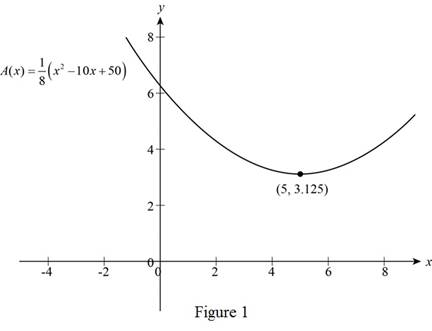# The function that models the total area enclosed by two squares.### Precalculus: Mathematics for Calcu...

6th Edition
Stewart + 5 others
Publisher: Cengage Learning
ISBN: 9780840068071### Precalculus: Mathematics for Calcu...

6th Edition
Stewart + 5 others
Publisher: Cengage Learning
ISBN: 9780840068071

#### Solutions

Chapter 2, Problem 24P

(a)

To determine

## To find: The function that models the total area enclosed by two squares.

Expert Solution

The function that models the area of both the squares is A(x)=18(x210x+50).

### Explanation of Solution

Given:

Length of wire is 10cm.

Length of first wire after cutting is x and length of second wire is (10x).

Calculation:

If length of wires are x and (10x) then length of side of square is x4 and (10x4).

Total enclosed area (A) by both the squares is,

(A)=a1+a2 (1)

Where, A is total enclosed area, a1 is area of square whose side is x4 and a2 is area of square whose side is (10x4).

Area of a square is,

Area=(side)(side)=ll (2)

Where, l is side of square.

Substitute x4 for l in above equation (2) for area a1,

a1=x4x4=x216

The area enclosed by square of side x4 is x216.

Substitute 10x4 for l in equation (2) for area a2,

a2=(10x4)(10x4)=10010x10x+x216=x220x+10016

The total area is calculated as follows,

A(x)=x216+x220x+10016=2x220x+10016=2(x210x+50)16=x210x+508

Further solving,

A(x)=18(x210x+50)

Thus, the function that models the area of both the squares is A(x)=18(x210x+50).

(b)

To determine

### To find: The value of x such that area enclosed by the two squares is minimum.

Expert Solution

The value of x that minimizes the area of the square is 5 cm.

### Explanation of Solution

Function that models the area of square as calculated in part (a) is A(x)=18(x210x+50).

Sketch the function A(x) using graphing calculator as shown below.From the above figure, it can be observed that the function has minimum value at x=5.

Therefore, for the area to be minimum the length of the first wire must be 5 cm.

### Have a homework question?

Subscribe to bartleby learn! Ask subject matter experts 30 homework questions each month. Plus, you’ll have access to millions of step-by-step textbook answers!